# Analysis of the Pied Flycatcher Data

#### 2018-01-28

As a first example of the functions in this package we will fit a model to the pied flycatcher data. We will start by fitting a model with fixed effects on the mean and variance components. Specifically, we will model the load returned on each trip by the adult flycatchers a independent normal random variables with mean given by a linear combination of the logarithm of the inter-visit interval (IVI), the broodsize manipulation (broodsize), and the adult’s sex, and standard deviation given by a linear combination of broodsize and sex, on the log scale. We will then incorporate individual random effects into this model.

# Library

First you have to load the package:

## Load package
library(dalmatian)

# Raw data

The raw data for this example is provided in the package and can be accessed with:

## Load pied flycatcher data
data(pied_flycatchers_1)

This data contains records on 5795 different food deliveries recorded during the pied flycatcher experiment. The response variable, load, records the load rounded to the nearest .1 gram. We want to model the logarithm of the load, and we also want to account for the rounding. To do this we will create two new variables in the data frame, lower and upper, which bound the logarithm of the true load. Not that we add .001 when the observed value is zero to avoid problems with the logarithm.

## Create variables bounding the true load
pfdata$lower=ifelse(pfdata$load==0,log(.001),log(pfdata$load-.049)) ## Warning in log(pfdata$load - 0.049): NaNs produced
pfdata$upper=log(pfdata$load+.05)

# Model 1: Fixed Effects

Our initial model for the logarithm of the load returned on the $$j$$th trip by adult $$i$$ will be $$N(\mu_{ij},\sigma_{ij}^2)$$ where: $\mu=\beta_0 + \beta_1 \mathrm{log(IVI)_{ij} + \beta_2 broodsize_i + \beta_3 sex_i}$ and $\log(\sigma)=\psi_0 + \psi_1 \mathrm{broodsize}_i + \psi_2\mathrm{sex}_i$.

## Model

The first step is to define the models for the mean and variance components. These models are simply lists with (up to) two named components, fixed and random, which provide the details on the fixed and random effects. Both lists must contain a variable name specifying the basename for the coefficients and a variable formula specifying the effects. The fixed list should also contain an object called priors which specifies the priors for coefficients in the fixed effects portion of the model. Random effects are currently assumed to be normal with mean zero and unknown variances which are assigned the half $$t$$-distribution with ? degrees of freedom and scale ?. The optional parameter link can also be used to specify a link function for either component.

The components of the model for the pied flycatcher data specified above would be generated with:

## Mean model
mymean=list(fixed=list(name="alpha",
formula=~ log(IVI) + broodsize + sex,
priors=list(c("dnorm",0,.001))))

## Variance model
myvar=list(fixed=list(name="psi",
formula=~broodsize + sex,
priors=list(c("dnorm",0,.001))))

These two objects will now be used to generate the JAGS code, data, and initial values for running the model.

## Running the Model with dalmatian

The primary function in the package dalmatian automates the creation of the JAGS code, data, and initial values and then passes these to JAGS via the functions in the rjags package. The dalmatian function takes requires several arguments including the data frame for the analysis, the model objects created above, and the name of the JAGS model file. It also requires two lists containing named arguments for the functions jags.model and coda.samples from the rjags package. Descriptions of the arguments for these two functions are available in their own help pages. Any arguments that are not specified in these lists will take the default values given by rjags. The two exceptions are the file argument of jags.model and the n.iter argument of coda.samples which do not have default values and must be specified. The number of parallel chains will be taken from the jags.model list. If this value is not specified then three chains will be run in order that convergence diagnostics can be computed. Note that the chains have been run for a relatively small number of iterations and heavily subsampled to satisfy the size requirements for packages on . Neither of these is recommended in practice.

## Set working directory
## By default uses a system temp directory. You probably want to change this.
workingDir <- tempdir()

## Define list of arguments for jags.model()

## Define list of arguments for coda.samples()
cs.args <- list(n.iter=5000,thin=20)

## Run the model using dalmatian
## This is how the model is run. However, to save you time we will load output from a previous run instead.
if(runModels){
pfresults <- dalmatian(df=pfdata,
mean.model=mymean,
variance.model=myvar,
jags.model.args=jm.args,
coda.samples.args=cs.args,
rounding=TRUE,
lower="lower",
upper="upper",
debug=FALSE)

save(pfresults,"pfresults.RData")
}
if(!runModels){
}

## Results

The function dalmatian returns list containing three objects: 1. jags.model.args The full list of arguments passed to jags.model. This includes the variables in the input list along with the name of the model file, the formatted data, and the initial values. 2. coda.samples.args The full list of arguments passed to coda.samples. This includes the variables in the input list along with the complete object returned by jags.model.args and the character vector including the names of the monitored parameters. 3. coda An object of class mcmc.list and length n.chains containing the samples generated by JAGS.

## Results

Inference is based on the output from the MCMC chain which is stored in the coda object. Summaries of the MCMC sampler and the posterior distribution can be constructed from coda with a variety of tools designed to work with objects of the mcmc.list class. For example, we can use the tools in the package coda to generate traceplots, density plots and histograms, numerical summaries of the posterior, and convergence diagnostics. The ggmcmc package can also be used to create fancier looking traceplots, density plots, and plots summarizing the posterior distribition in terms of posterior means and quantiles (credible intervals). However, several wrapper functions exist within the package to automate some of these tasks.

The first thing we should do is to check the convergence of the chains. Summary statistics computed from the output in coda are only valid if the chain has converged and is sampling from the correct distribution. The wrapper function convergence() applies three of the convergence diagnostics from the coda package.

## Compute convergence diagnostics
pfconvergence <- convergence(pfresults)

## Gelman-Rubin diagnostics
pfconvergence$gelman ## Potential scale reduction factors: ## ## Point est. Upper C.I. ## alpha.(Intercept) 1 1.00 ## alpha.log(IVI) 1 1.00 ## alpha.broodsize 1 1.01 ## alpha.sex 1 1.01 ## psi.(Intercept) 1 1.02 ## psi.broodsize 1 1.01 ## psi.sex 1 1.01 ## Raftery diagnostics pfconvergence$raftery
##
## Quantile (q) = 0.025
## Accuracy (r) = +/- 0.005
## Probability (s) = 0.95
##
## You need a sample size of at least 3746 with these values of q, r and s
## Effective sample size
pfconvergence$effectiveSize ## alpha.(Intercept) alpha.log(IVI) alpha.broodsize alpha.sex ## 750.000 680.420 750.000 750.000 ## psi.(Intercept) psi.broodsize psi.sex ## 1242.813 750.000 1095.642 Details of these measures can be found in the help files of the coda package. Not surprisingly, these all suggest that the chains need to be run for longer. Convergence and mixing of the chains can also be examined visually through traceplots. The wrapper function traceplots generates traces using the functions from the ggmcmc package and separates the variables by the model components. One of the advantages of using ggmcmc which is based on ggplot2 is that the plots can be saved and easily reproduced. Here I have thinned the chains further prior to plotting to reduce the size of the vignette. Note that thinning is conducted relative to the original chain, not the already thinned chain. I.e., thinning by 100 selects the output from every 100th iteration of the original chain. ## Generate traceplots pftraceplots <- traceplots(pfresults,plot=FALSE,nthin=100) ## Fixed effects for mean pftraceplots$meanFixed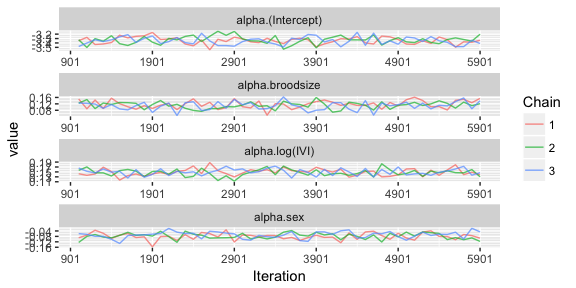## Fixed effects for variance
pftraceplots$varianceFixed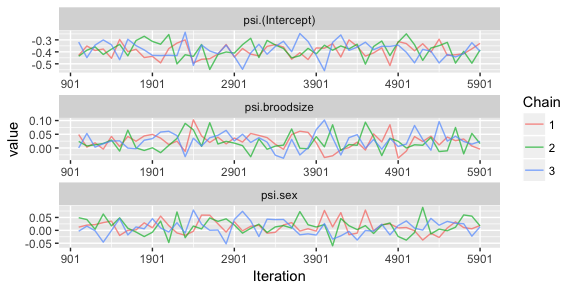Numerical summaries of the posterior distribution computed from the output in coda can be obtained with the summary function. ## Compute numerical summaries summary(pfresults) ## ## Iterations = 1001:5981 ## Thinning interval = 20 ## Number of chains = 3 ## Sample size per chain = 250 ## ## Posterior Summary Statistics for Each Model Component ## ## Mean Model: Fixed Effects ## Mean Median SD Lower 95% Lower 50% Upper 50% Upper 95% ## (Intercept) -3.34 -3.34 0.08 -3.50 -3.39 -3.28 -3.18 ## log(IVI) 0.15 0.15 0.01 0.13 0.14 0.16 0.17 ## broodsize 0.11 0.11 0.03 0.06 0.10 0.13 0.16 ## sex -0.07 -0.07 0.02 -0.12 -0.10 -0.06 -0.03 ## ## Variance Model: Fixed Effects ## Mean Median SD Lower 95% Lower 50% Upper 50% Upper 95% ## (Intercept) -0.39 -0.39 0.06 -0.50 -0.44 -0.35 -0.25 ## broodsize 0.02 0.02 0.03 -0.04 0.00 0.04 0.07 ## sex 0.01 0.01 0.03 -0.04 0.00 0.04 0.07 For each component of the model the tables provide statistics including the estimated posterior mean, median, and standard deviation and the end points of the 50% and 95% highest posterior density credible intervals. Note that these summaries are only valid if the previous diagnostics have suggested that the chain has converged and the effective sample size is large enough. Graphical summaries can also be computed with the caterpillar which, like traceplots, is a simple wrapper for the functions in the ggmcmc package. ## Generate caterpillar pfcaterpillar <- caterpillar(pfresults,plot = FALSE) ## Fixed effects for mean pfcaterpillar$meanFixed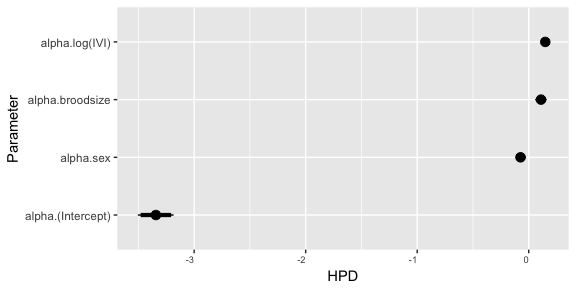## Fixed effects for variance
pfcaterpillar$varianceFixed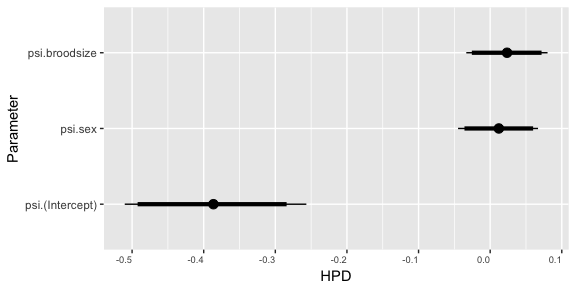# Model 2: Random Effects We will now consider adding individual (bird) random effects to both the fixed and random components of our model. This can be done by simply adding to the model components created above and then rerunnig the model. The new model will have mean and variance $\mu=\beta_0 + \beta_1 \mathrm{log(IVI)_{ij} + \beta_2 broodsize_i + \beta_3 sex_i + \epsilon_{i}}$ and $\log(\sigma)=\psi_0 + \psi_1 \mathrm{broodsize}_i + \psi_2\mathrm{sex}_i + \xi_j$ where $$\epsilon_i \sim N(0,\tau^2_\mu)$$ and $$\xi_i \sim N(0,\tau^2_\sigma)$$. By default the variances $$\tau^2_\epsilon$$ and $$\tau^2_\xi$$ are assigned half-$$t$$ prior distributions. ## Model Instead of creating new model objects we can simply add to the old objects by creating the random fields. As with the fixed effects, these fields are lists which must include a basename for the random effects and formula specifying the random effects model. # Random component of mean mymean$random=list(name="epsilon",formula=~-1 + indidx)

# Random component of variance
fixed.variance = tail(pfresults$coda[[i]],1)[5:7], sd.mean = 1, sd.variance=1) }) ## Define list of arguments for jags.model() jm.args <- list(file=file.path(workingDir,"pied_flycatcher_2_jags.R"),inits=inits,n.adapt=1000) ## Define list of arguments for coda.samples() cs.args <- list(n.iter=5000,thin=10) ## Run the model using dalmatian ## This is how the model is run. However, to save you time we will load output from a previous run instead. if(runModels){ pfresults2 <- dalmatian(df=pfdata, mean.model=mymean, variance.model=myvar, jags.model.args=jm.args, coda.samples.args=cs.args, rounding=TRUE, lower="lower", upper="upper", debug=FALSE) save(pfresults2,"pfresults2.RData") } if(!runModels){ load(system.file("Pied_Flycatchers_1","pfresults2.RData",package="dalmatian")) } ## Results As before, we can use the wrapper functions within the package to examine the convergence and compute summaries of the posterior distribution. 1. Convergence diagnostics ## Compute convergence diagnostics pfconvergence2 <- convergence(pfresults2) ## Gelman-Rubin diagnostics pfconvergence2$gelman
## Potential scale reduction factors:
##
##                   Point est. Upper C.I.
## alpha.(Intercept)       1.01       1.04
## alpha.log(IVI)          1.00       1.00
## alpha.broodsize         1.01       1.03
## alpha.sex               1.01       1.03
## psi.(Intercept)         1.00       1.00
## psi.broodsize           1.01       1.03
## psi.sex                 1.00       1.02
## sd.epsilon.indidx       1.08       1.11
## sd.epsilon.indidx       1.08       1.11
## sd.xi.indidx            1.01       1.02
## Raftery diagnostics
pfconvergence2$raftery ## ## Quantile (q) = 0.025 ## Accuracy (r) = +/- 0.005 ## Probability (s) = 0.95 ## ## You need a sample size of at least 3746 with these values of q, r and s ## Effective sample size pfconvergence2$effectiveSize
## alpha.(Intercept)    alpha.log(IVI)   alpha.broodsize         alpha.sex
##          654.0340          829.4722          511.8398          594.6217
##   psi.(Intercept)     psi.broodsize           psi.sex sd.epsilon.indidx
##          790.6854          688.6121          704.4285          558.6042
## sd.epsilon.indidx      sd.xi.indidx
##          558.6042          565.8051
1. Traceplots
## Generate traceplots
pftraceplots2 <- traceplots(pfresults2,plot = FALSE,nthin=100)

## Fixed effects for mean
pftraceplots2$meanFixed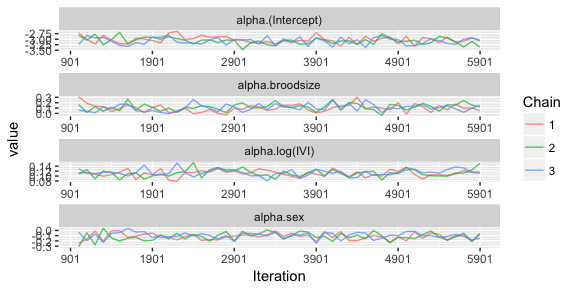## Fixed effects for variance pftraceplots2$varianceFixed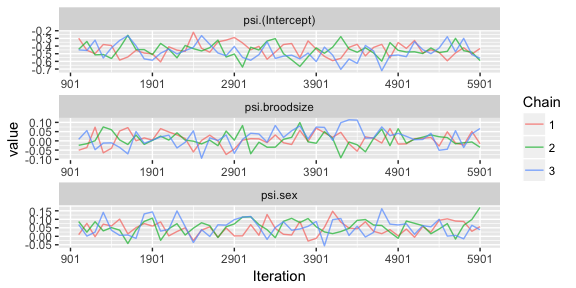## Random effects variances for mean
pftraceplots2$meanRandom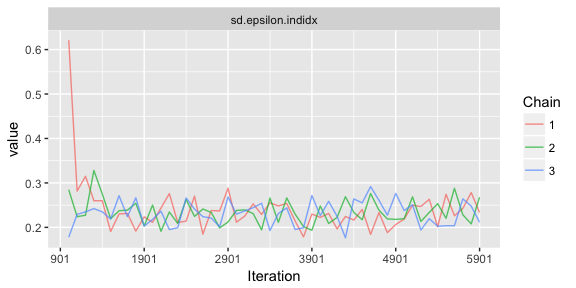## Random effects variances for variances pftraceplots2$varianceRandom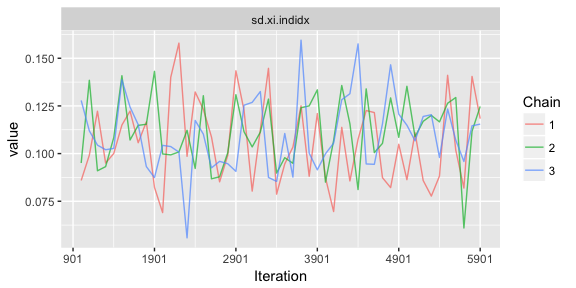1. Numerical summaries
## Compute numerical summaries
summary(pfresults2)
##
## Iterations = 1001:5981
## Thinning interval = 20
## Number of chains = 3
## Sample size per chain = 250
##
## Posterior Summary Statistics for Each Model Component
##
## Mean Model: Fixed Effects
##              Mean Median   SD Lower 95% Lower 50% Upper 50% Upper 95%
## (Intercept) -3.08  -3.09 0.17     -3.37     -3.16     -2.94     -2.70
## log(IVI)     0.11   0.11 0.01      0.09      0.10      0.12      0.14
## broodsize    0.11   0.12 0.07     -0.03      0.06      0.15      0.24
## sex         -0.10  -0.10 0.07     -0.25     -0.14     -0.04      0.03
##
## Mean Model: Random Effects
##        Mean Median   SD Lower 95% Lower 50% Upper 50% Upper 95%
## indidx 0.23   0.23 0.04      0.18      0.21      0.24      0.29
##
## Variance Model: Fixed Effects
##              Mean Median   SD Lower 95% Lower 50% Upper 50% Upper 95%
## (Intercept) -0.45  -0.45 0.10     -0.63     -0.53     -0.41     -0.26
## broodsize    0.01   0.00 0.04     -0.08     -0.02      0.03      0.09
## sex          0.05   0.05 0.04     -0.03      0.03      0.09      0.13
##
## Variance Model: Random Effects
##        Mean Median   SD Lower 95% Lower 50% Upper 50% Upper 95%
## indidx 0.11   0.11 0.02      0.07      0.09      0.12      0.16
1. Graphical summaries
## Generate caterpillar
pfcaterpillar2 <- caterpillar(pfresults2,plot = FALSE)

## Fixed effects for mean
pfcaterpillar2$meanFixed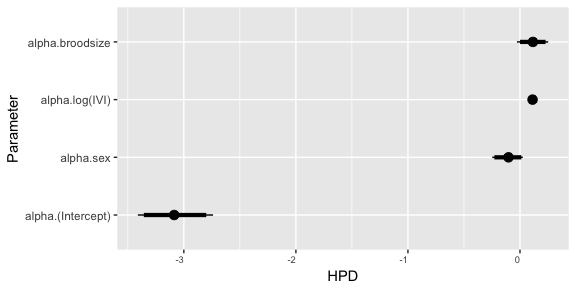## Fixed effects for variance pfcaterpillar2$varianceFixed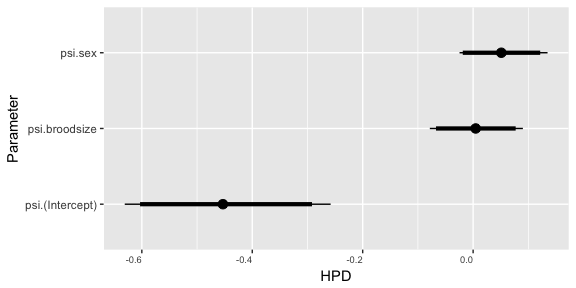## Predictions

By using ‘prediction’ function, predictions for the mean and variance models can be calculated. The ‘prediction’ function should take a data frame including all variables required and their names defined for the usage of ‘dalmatian’ function. For the Pied Flycatcher data, previously ‘log(IVI)’ was used to define the mean and variance models. Hence, it should be included in the data frame as following:

## Add 'log(IVI)' variable in pfdata
pfdata$'log(IVI)' <- log(pfdata$IVI)

We can now get predictions.

## Plot predictions for the Model 1
pred.pfresults <- fitted(object = pfresults,
df = pfdata,
method = "mean",
ci = TRUE,
level = 0.95)

# predictions for the Model 2
pred.pfresults2 <- fitted(object = pfresults2,
df = pfdata,
method = "mean",
ci = TRUE,
level = 0.95)